# Potential field

(diff) ← Older revision | Latest revision (diff) | Newer revision → (diff)

The vector field generated by the gradients of a scalar functionin several variables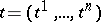which belong to some domainin an-dimensional space. The functionis called the scalar potential (potential function) of this field. A potential field is completely integrable over: The Pfaffian equation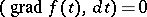has the level lines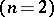or the level surfaces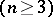of the potentialas-dimensional integral manifolds. Any regular covariant fieldthat is completely integrable overis obtained by multiplying the potential field by a scalar:The scalar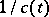is called an integrating factor of the Pfaffian equation. The following equalities serve as a test for the field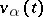to be the gradient of a potential (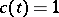):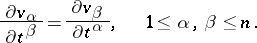They indicate that the fieldis rotation free (see Curl).

The concept of a potential field is widely used in mechanics and physics. The majority of force fields and electric fields can be considered as potential fields. For instance, if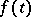is the pressure at a pointof an ideal fluid filling a region, then the vector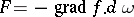is equal to the equilibrium pressure force applied to the volume element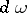. Ifis the temperature of a heated bodyat a point, then the vector, whereis the thermal conductivity coefficient, is equal to the density of the heat flow in the direction of less heated parts of the body (in the direction orthogonal to the isothermal surfaces).

In the above, complete integrability of a vector field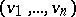means that the Pfaffian equationdefines an involutive distribution, i.e. an integrable one. A differential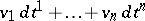such thatfor some potentialis called a total differential and the corresponding functionis sometimes called a complete integral. Especially for,is called an exact differential equation.